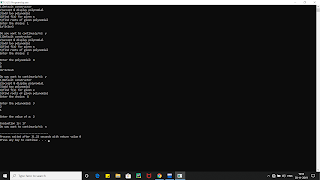### Implement a class Quadratic that represents degree two polynomials i.e., polynomials of type ax2+bx+c. The class will require three data members corresponding to a, b and c. Implement the following operations: 1. A constructor (including a default constructor which creates the 0 polynomial). 2. Overloaded operator+ to add two polynomials of degree 2. 3. Overloaded << and >> to print and read polynomials. To do this, you will need to decide what you want your input and output format to look like. 4. A function eval that computes the value of a polynomial for a given value of x. 5. A function that computes the two solutions of the equation ax2+bx+c=0.

Implement a class Quadratic that represents degree two polynomials i.e., polynomials of type
ax2+bx+c. The class will require three data members corresponding to a, b and c. Implement
the following operations:
1. A constructor (including a default constructor which creates the 0 polynomial).
3. Overloaded << and >> to print and read polynomials. To do this, you will need to
decide what you want your input and output format to look like.
4. A function eval that computes the value of a polynomial for a given value of x.
5. A function that computes the two solutions of the equation ax2+bx+c=0.OUTPUT FOR THE PROGRAM
#include<iostream>
#include<cmath>
using namespace std;
class polynomial
{
int a,b,c;
public:
polynomial()
{
a=b=c=0;
}
polynomial(int x,int y,int z)
{
a=x;
b=y;
c=z;
}
polynomial operator +(polynomial T)
{
polynomial R;
R.a=a+T.a;
R.b=b+T.b;
R.c=c+T.c;
return R;
}
friend istream &operator >>(istream &IN,polynomial &T)
{
IN>>T.a>>T.b>>T.c;
return IN;
}
friend ostream &operator <<(ostream &OUT,polynomial &T)
{
OUT<<T.a<<"x^2+"<<T.b<<"x+"<<T.c;
return OUT;
}
void eval(polynomial T,int x)
{
int eval;
eval=T.a*x*x+T.b*x+T.c;
cout<<"\nEvaluation is: "<<eval;
}
void compute(polynomial T)
{
int x,r1,r2;
x=T.b*T.b-4*T.a*T.c;
if(x<0)
{
cout<<"\nRoots are real & distinct";
r1=-T.b+sqrt(x)/2*T.a;
r2=-T.b-sqrt(x)/2*T.a;
cout<<"\nRoots are: "<<r1 <<"&"<<r2;
}
else if(x=0)
{
cout<<"\nRoots are real & same";
r1=-T.b/2*T.a;
cout<<"\nRoots are: "<<r1 <<"&"<<r1;
}
else
cout<<"\nRoots are complex";
}
};
int main()
{
int choice,x;
char con;
polynomial s1(1,2,3),s2,s3;
do
{
cout<<"1)Default constructor\n2)accept & display polynomial\n3)add two polynomial\n4)find f(x) for given x\n5)find roots of given polynomial";
cout<<"\nEnter the choice: ";
cin>>choice;
switch(choice)
{
case 1:
{
cout<<s1<<endl;
break;
}
case 2:
{
cout<<"\nEnter the polynomial: ";
cin>>s2;
cout<<s2<<endl;
break;
}
case 3:
{
cout<<"\nEnter 1st Polynomial: ";
cin>>s1;
cout<<"\nEnter 2nd Polynomial: ";
cin>>s2;
s3=s1+s2;
cout<<s3<<endl;
break;
}
case 4:
{
cout<<"\nEnter the polynomial: ";
cin>>s1;
cout<<"\nEnter the value of x: ";
cin>>x;
s1.eval(s1,x);
break;
}
case 5:
{
cout<<"\nEnter the polynomial: ";
cin>>s1;
s1.compute(s1);
break;
}
}
cout<<"\nDo you want to continue(y/n): ";
cin>>con;
}
while(con!='n');
return 0;
}